Ex 14.1

Chapter 14 Class 10 Statistics
Serial order wise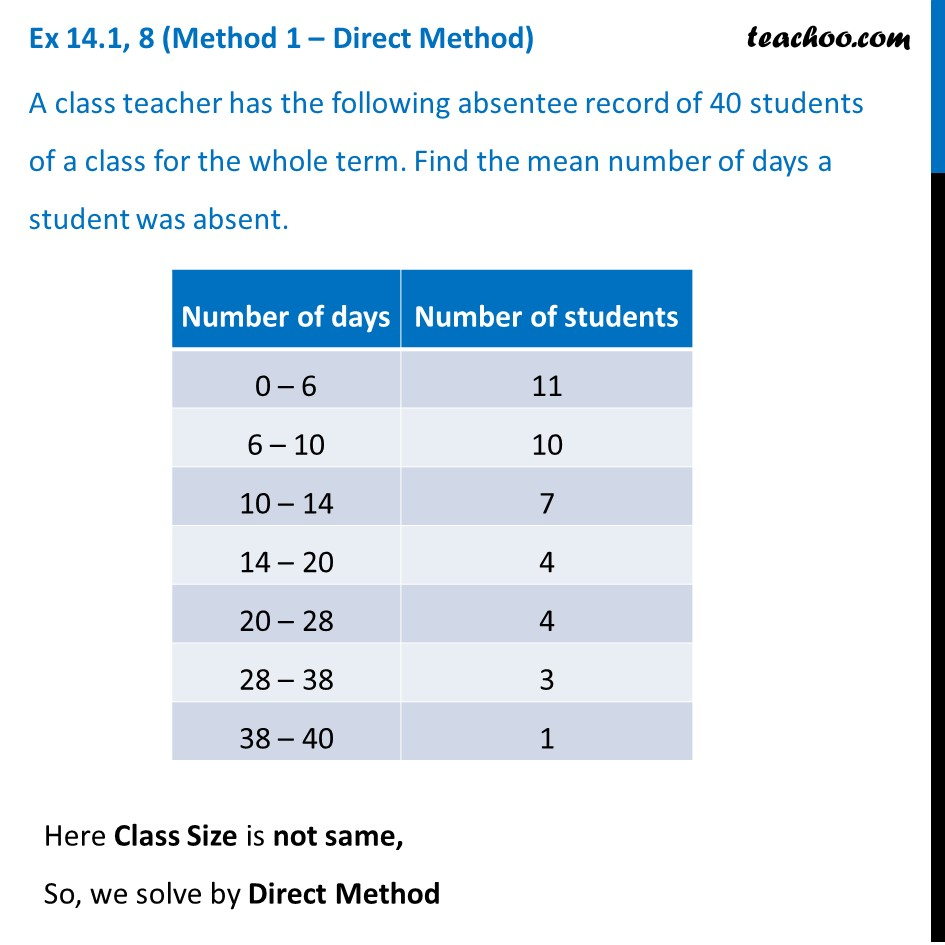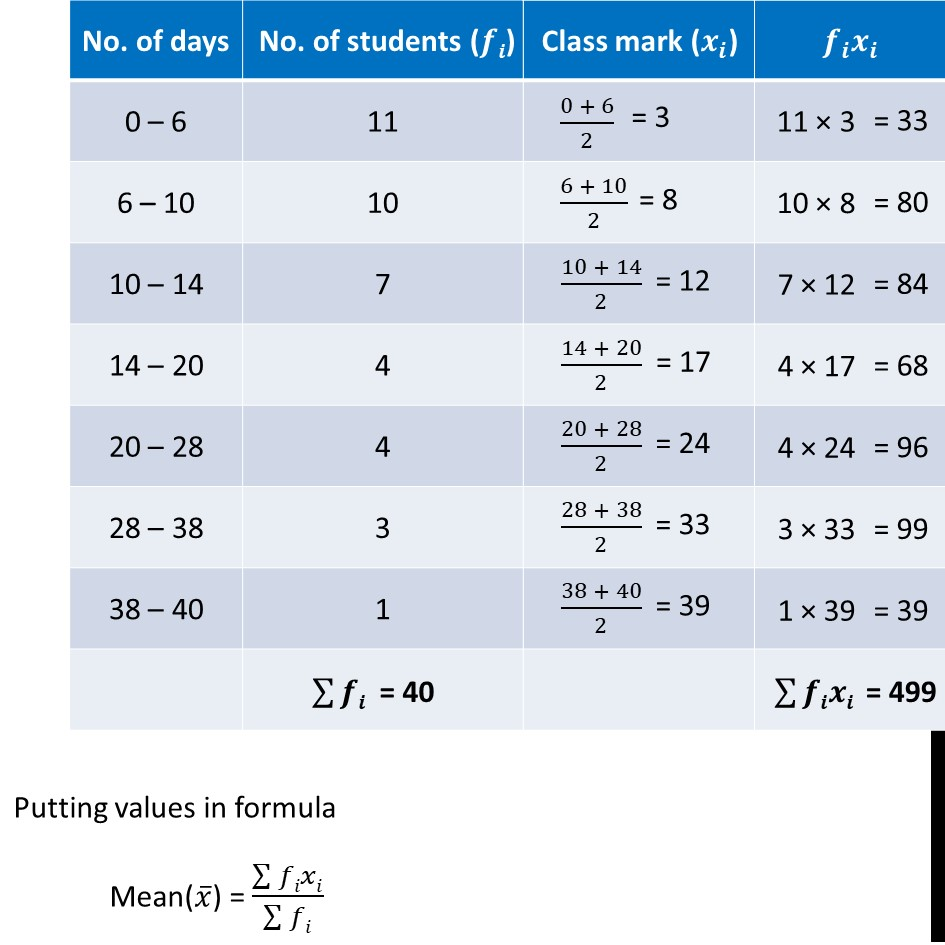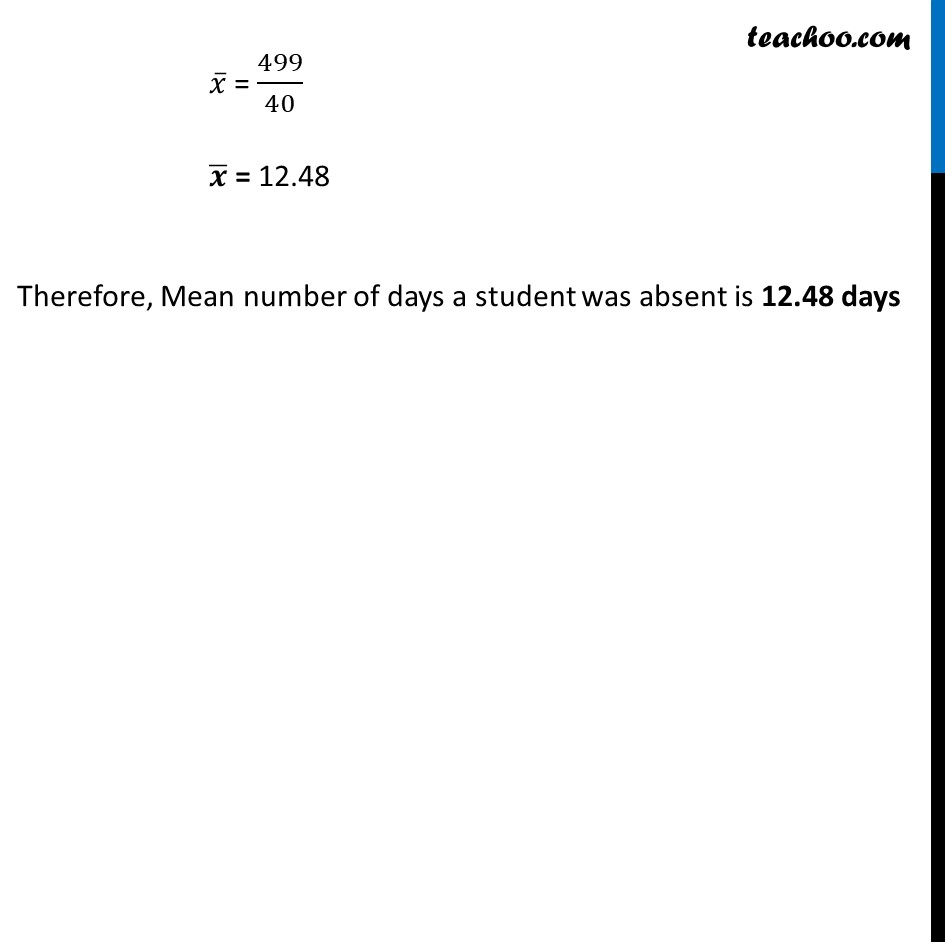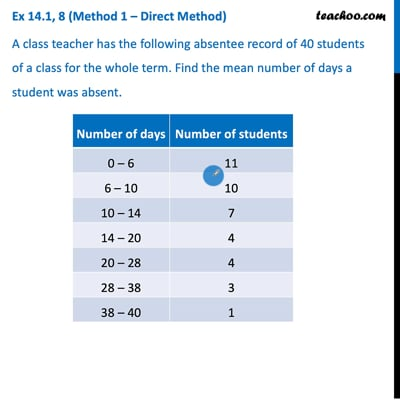This video is only available for Teachoo black users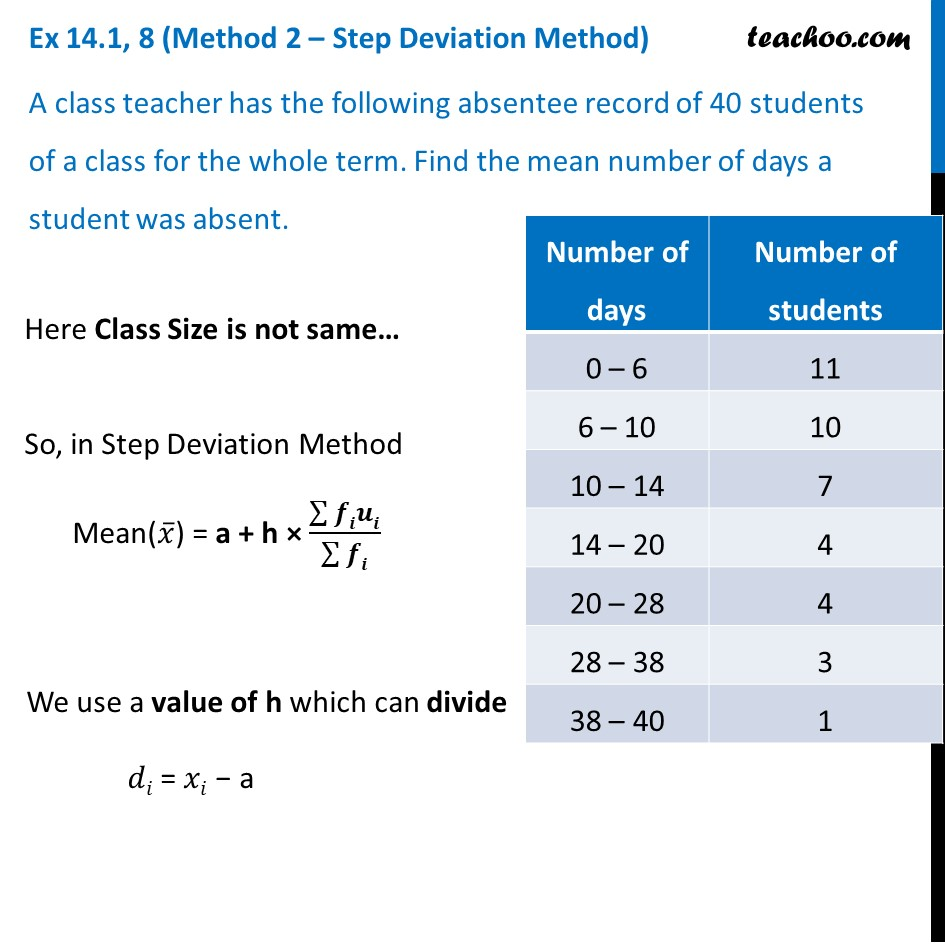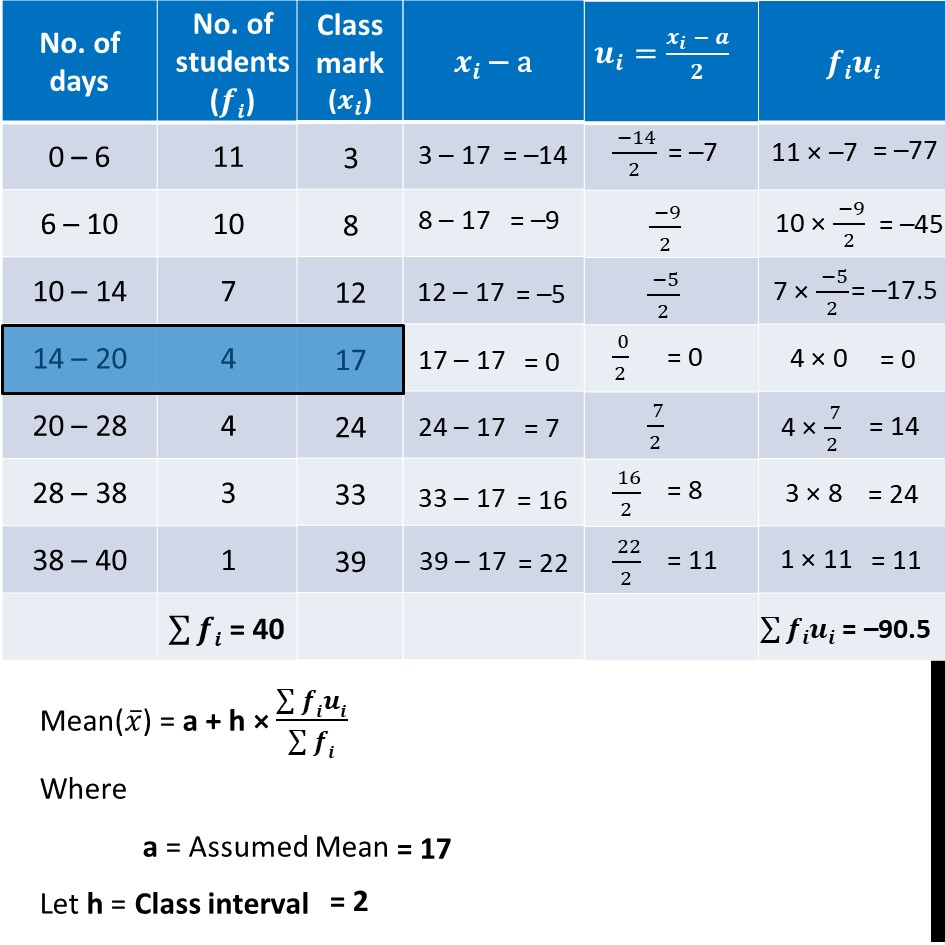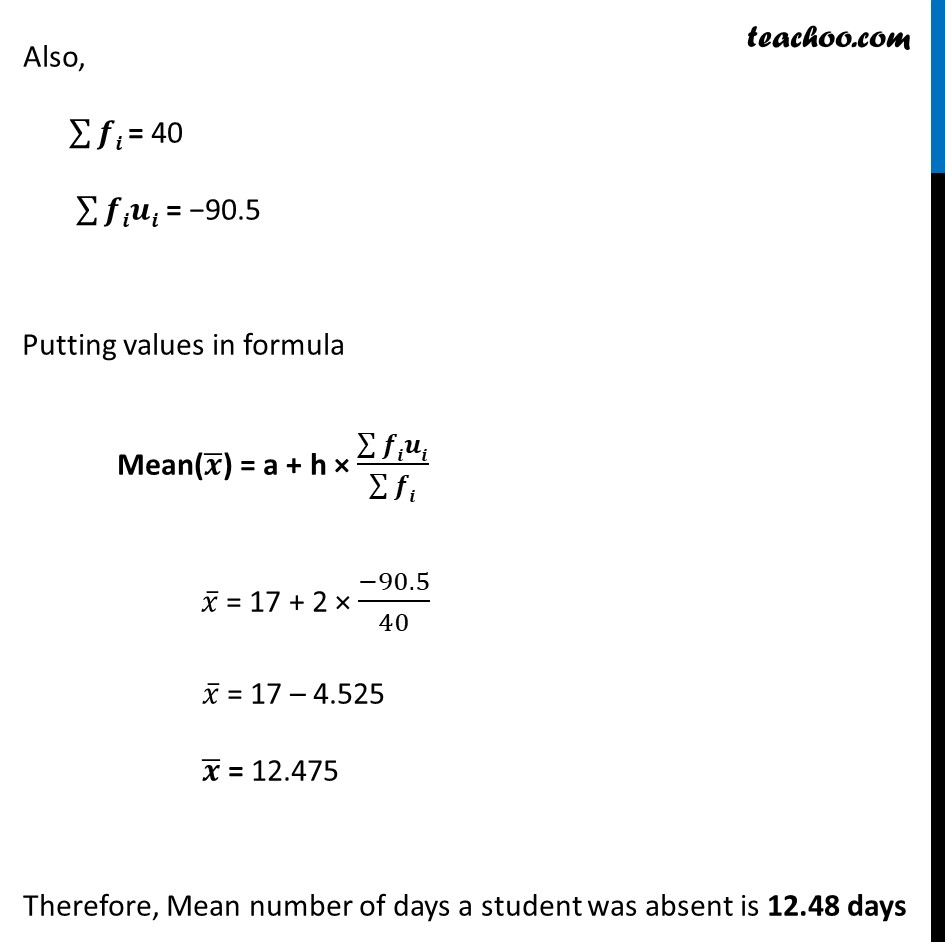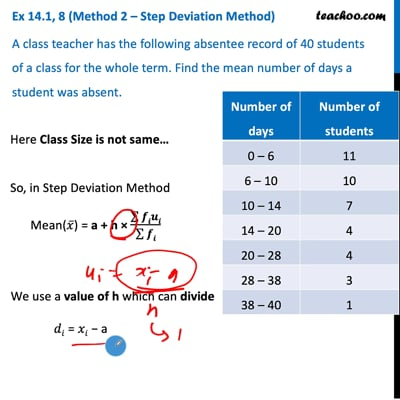This video is only available for Teachoo black users

Solve all your doubts with Teachoo Black (new monthly pack available now!)

### Transcript

Ex 14.1, 8 (Method 1 – Direct Method) A class teacher has the following absentee record of 40 students of a class for the whole term. Find the mean number of days a student was absent. Here Class Size is not same, So, we solve by Direct Method Putting values in formula Mean(𝑥 ̅) = (∑▒𝑓𝑖𝑥𝑖)/(∑▒𝑓𝑖) 𝑥 ̅ = 499/40 𝒙 ̅ = 12.48 Therefore, Mean number of days a student was absent is 12.48 daysEx 14.1, 8 (Method 2 – Step Deviation Method) A class teacher has the following absentee record of 40 students of a class for the whole term. Find the mean number of days a student was absent. Here Class Size is not same… So, in Step Deviation Method Mean(𝑥 ̅) = a + h × (∑▒𝒇𝒊𝒖𝒊)/(∑▒𝒇𝒊) We use a value of h which can divide 𝑑𝑖 = 𝑥𝑖 − a Mean(𝑥 ̅) = a + h × (∑▒𝒇𝒊𝒖𝒊)/(∑▒𝒇𝒊) Where a = Assumed Mean Let h = Class interval Also, ∑▒𝒇𝒊 = 40 ∑▒𝒇𝒊𝒖𝒊 = −90.5 Putting values in formula Mean(𝒙 ̅) = a + h × (∑▒𝒇𝒊𝒖𝒊)/(∑▒𝒇𝒊) 𝑥 ̅ = 17 + 2 × (−90.5)/40 𝑥 ̅ = 17 – 4.525 𝒙 ̅ = 12.475 Therefore, Mean number of days a student was absent is 12.48 days